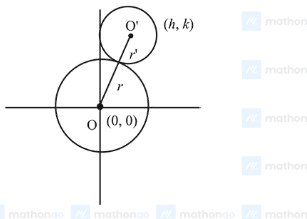# The locus of the centres of the circles,

Question:

The locus of the centres of the circles, which touch the circle, $x^{2}+y^{2}=1$ externally, also touch the $y$-axis and lie in the first quadrant, is:

1. (1) $x=\sqrt{1+4 y}, y \geq 0$

2. (2) $y=\sqrt{1+2 x}, x \geq 0$

3. (3) $y=\sqrt{1+4 x}, x \geq 0$

4. (4) $x=\sqrt{1+2 y}, y \geq 0$

Correct Option: 2,

Solution:

Let centre of required circle is $(h, k)$.

$\therefore \mathrm{OO}^{\prime}=r+r^{\prime} \quad$ [By the diagram]

$\Rightarrow \sqrt{h^{2}+k^{2}}=1+h$

$\Rightarrow h^{2}+k^{2}=1+h^{2}+2 h$

$\Rightarrow k^{2}=1+2 h$

$\therefore$ locus is $y=\sqrt{1+2 x}$

$x \geq 0$